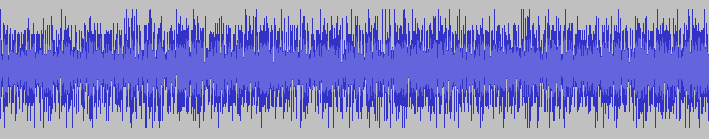# What Does Perlin Noise Sound Like?In one of the previous posts, we discussed how to use Perlin noise to generate some imagery. This time, I wanted to try something slightly different: I was curious what it would be like to generate an audio signal (as opposed to pictures) with Perlin noise.

## The Noise Function

Since audio is a one-dimensional signal, the one-dimensional version of the noise function should fit our purposes nicely. With some very minor changes, it's just a copy of the code from the previous post (we're going to be using C++ here, while the original version was meant to be used in a GLSL shader). Anyway, here's the code we're going to be using to generate noise:

``````
float fade(float t) {
return t*t*t*(t*(t*6.0f - 15.0f) + 10.0f);
}

float grad(float p) {
static float rand_noise;
static bool have_noise = false;
if (!have_noise) {
srand(time(NULL));
for (size_t i = 0; i < sizeof(rand_noise) / sizeof(float); ++i) {
rand_noise[i] = static_cast(rand())/static_cast(RAND_MAX);
}
have_noise = true;
}
float v =  rand_noise[static_cast(floor(p)) % (sizeof(rand_noise)/sizeof(float))];
return v > 0.5f ? 1.0f : -1.0f;
}

float noise(float p) {
float p0 = floor(p);
float p1 = p0 + 1.0f;

float t = p - p0;
float fade_t = fade(t);

float g0 = grad(p0);
float g1 = grad(p1);

return (1.0f - fade_t)*g0*(p - p0) + fade_t*g1*(p - p1);
}
```
```

The main difference is how we generate randomness for the gradients (GLSL version used a noise texture).

## Generating Sound

We're going to be writing a stream of raw PCM samples to a file, using 44.1 kHz sampling rate (meaning 1 second worth of sound will contain 44100 PCM samples). We shall be using signed 16-bit samples. The code is actually quite straighforward:

``````
int main() {
const int duration_seconds = 60;
const int sampling_rate_hz = 44100;
const int freq = 440;

FILE * output = fopen("output.pcm", "wb");
for (size_t sample_idx = 0;
sample_idx < duration_seconds * sampling_rate_hz;
++sample_idx) {

/* The expression below defines how noise function samples are distributed
across seconds. We want each second to contain `freq' samples of noise at
integer points. */
float x1 =
static_cast(sample_idx) / (static_cast(sampling_rate_hz) / static_cast(freq));

float n = noise(x1);
float max = std::numeric_limits::max();
float min = std::numeric_limits::min();
float range = max - min;
float s = range * n;
int16_t pcm_sample = s;
fwrite(&pcm_sample, sizeof(int16_t), 1, output);
}
fclose(output);
return 0;
}
```
```

If you compile and run the code, it will generate a file called "output.pcm", containing raw pcm samples. You can play it in Audacity. To do so, in Audacity, click File -> Import -> Raw Data, select the output file, ensure that the import dialog has encoding set to "Signed 16 bit PCM", number of channels set to 1 and that the sampling rate is set to 44100 Hz.

Alternatively, you can simply listen to the result here:

Of course, it is easy to create fractal noise by simply adding noise sampled at different frequencies together:

``````
int main() {
const int duration_seconds = 60;
const int sampling_rate_hz = 44100;
const int freq = 110;
const int freq2 = 220;
const int freq3 = 440;

FILE * output = fopen("output.pcm", "wb");
for (size_t sample_idx = 0;
sample_idx < duration_seconds * sampling_rate_hz;
++sample_idx) {
float x1 = static_cast(sample_idx) / (static_cast(sampling_rate_hz) / static_cast(freq));
float x2 = static_cast(sample_idx) / (static_cast(sampling_rate_hz) / static_cast(freq2));
float x3 = static_cast(sample_idx) / (static_cast(sampling_rate_hz) / static_cast(freq3));
float n = noise(x1);
float n2 = noise(x2);
float n3 = noise(x3);
float max = std::numeric_limits::max();
float min = std::numeric_limits::min();
float range = max - min;
float s = range * (0.5 * n + 0.3 * n2 + 0.2 * n3);
int16_t pcm_sample = s;
fwrite(&pcm_sample, sizeof(int16_t), 1, output);
}
fclose(output);
return 0;
}
```
```

And here's the result of that:

Compare the above to white noise and note how starkly different it sounds:

Just like its visual representation, white noise sounds synthetic, while Perlin noise sounds more natural. I find it similar to the sound you hear in the cabin of a flying plane, or maybe the muffled sound of traffic you hear from the top floor of a high-rise building.

I actually find this relaxing to listen to. Recommend putting it on to drown out the voices of people talking in the office to help you concentrate :-)

Like this post? Follow this blog on Twitter for more!